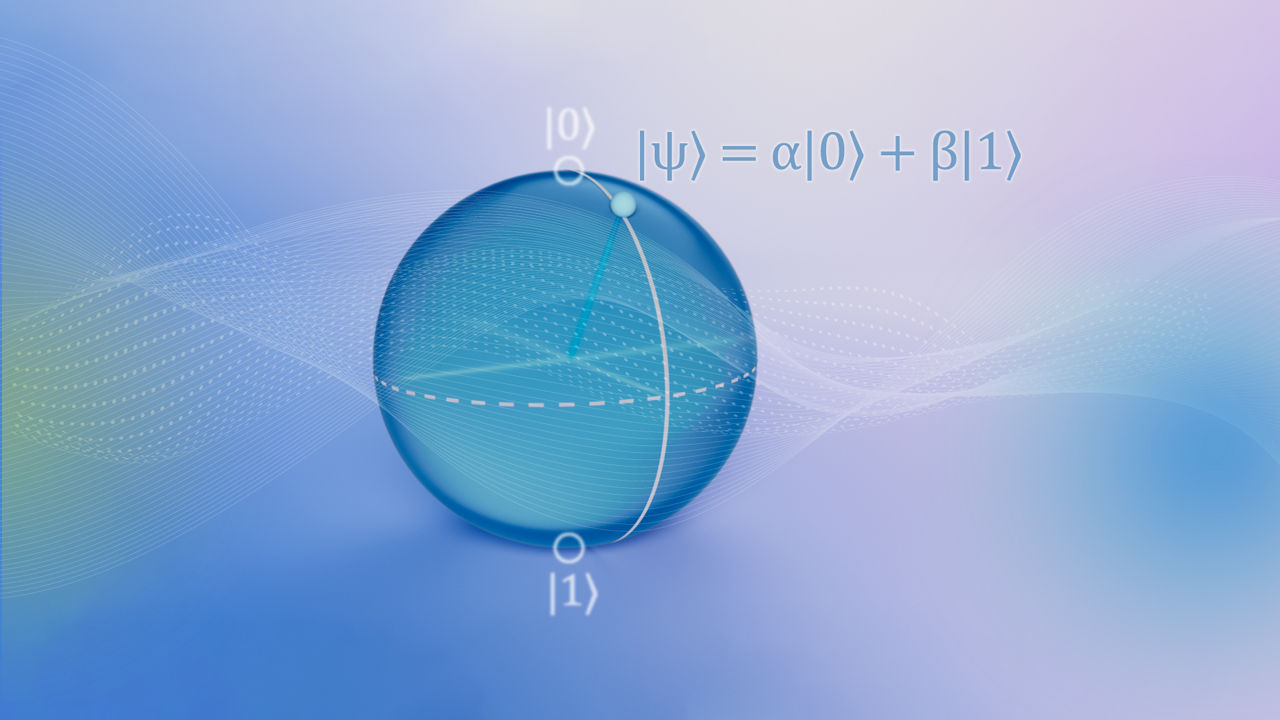# Explore quantum

## Superposition

Superposition is a fundamental concept in quantum mechanics, describing the condition in which a quantum system can exist in multiple states or configurations simultaneously. Classical bits can exist in two possible states, typically labeled as "0" and "1". In contrast, because a qubit is a quantum mechanical system, it can exist in the “0” state, the “1” state, or any state that is a linear combination of 0 and 1.

Mathematically, superposition is a linear combination of "0" and "1" and can be written as:

|ψ⟩ = α|0⟩ + β|1⟩

where |ψ⟩ is the state of the qubit, |0⟩ and |1⟩ are the basis states (or the computational basis states), and α and β are complex numbers called probability amplitudes. The probability amplitudes determine the probability of measuring the qubit in either state when a measurement is made.Importantly, the state of superposition can be maintained only while a quantum system is unobserved. Once measured, the wave function of a quantum system in a state of superposition "collapses" into one of the basis states. For a concrete example of how this might work if superposition could exist in the everyday world, imagine that a coin that is flipped and lands on a table. In classical mechanics -- and in the everyday world as we know it -- the coin ends up in a state of either heads or tails. In a quantum mechanical system, the coin could be both heads and tails at the same time, but only until someone or something observes it or measures it. In this analogy, once observed, the coin would take on the state of either heads or tails.

Superposition is a fundamental attribute of quantum computing systems. One of its benefits or uses is that it allows for the possibility of quantum parallelism. Because classical bits can be in only one of two possible states, 0 or 1, classical computers can perform only one computation at a time, e.g., “if the bit is a 1 do this, if not do that, next do this, etc.” In contrast, because a qubit can be in a superposition of 0 and 1, the quantum computer can perform multiple computations in parallel by processing all possible states of the qubits at once.

### Suggested Topics

#### Entanglement

Learn how quantum entanglement is used to enable parallelism in quantum computers, unlocking simultaneous calculation

#### Interference

Learn how quantum interference is used in quantum computation to amplify certain outcomes and suppress others

#### Single qubit gates

Learn how single qubit gates are used to form the building blocks for complex quantum circuits

#### Multi-qubit gates

Learn how multi-qubit gates are used in quantum computing to entangle qubits and manipulate qubit states